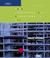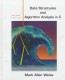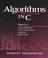Algorithms and Data Structures

Course Description

Building on the knowledge obtained in Programming and software engineering, basic data structures and algorithms are introduced. After the topic of dynamic memory allocation, function calling mechanism is presented. Computational complexity of algorithms is introduced. Search methods are presented, followed by recursion. Algorithms for sorting are explained and illustrated. Dynamic data structures are introduced: singly and multiply linked lists. Basic data structures, stack and queue, are presented followed by hashing, binary trees, and heap. Heap sort illustrates priority queue application.

General Competencies

Students will be able to design and program algorithms over advanced data structures, using the basics of the object-oriented program paradigm in C.

Learning Outcomes

1. describe the usage of various data structures
2. recognize the complexity of operations and algorithms
3. apply appropriate data structures and algorithms in solving real-life problems
4. develop computer programs to implement appropriate data structures and algorithms
5. assess the complexity of algorithms and computer programs
6. identify appropriate data structures and algorithms in solving real-life problems

Forms of Teaching

Lectures

Semester is organized in two cycles in totally 15 weeks, which accounts for two weeks for intermediate exams. There are four hours of lectures per week, divided in two terms of two hours (2+2).

Exams

There are two exams: midterm exam and final exam.

Laboratory Work

Two to three cycles of laboratory exercises.

Continuous Assessment Exam
Type Threshold Percent of Grade Threshold Percent of Grade
Laboratory Exercises 0 % 30 % 0 % 30 %
Mid Term Exam: Written 40 % 30 % 0 %
Final Exam: Written 50 % 40 %
Exam: Written 50 % 70 %
Comment:

On midterm and final exams students are required to gather the sum of at least 35 points.

Week by Week Schedule

1. Introduction. Review of basic programming and data structures. Memory allocation.
2. Function call mechanisms. Definition of algorithm. Complexity of algorithms.
3. Searching: sequential, jump-search, binary search.
4. Recursion.
5. Recursion examples, exercises.
6. Sorting algorithms: selection sort, bubble sort, insertion sort, Shell sort, mergesort, quicksort.
7. Linear list. Multiple linear lists.
8. Mid-term exam.
9. Mid-term exam.
10. Stack. Queue.
11. Hashing.
12. Hashing examples.
13. Introduction to graphs. Trees.
14. Heap.
15. Heap sort.

Study Programmes

Electrical Engineering and Information Technology and Computing (study)
(2. semester)

LiteratureAdam Drozdek (2000.), Data Structures and Algorithms in C++, Course TechnologyM. A. Weiss (1996.), Data Structures and Algorithm Analysis in C (2nd Edition), Addison WesleyR. Sedgewick (2001.), Algorithms in C: Fundamentals, Data Structures, Sorting, Searching and Graph Algorithms in C, Addison Wesley
Thomas H. Cormen, Charles E. Leiserson, Ronald L. Rivest, Clifford Stein (2009.), Rent eTextbook Buying Options Add To Cart - hardcover Add To Cart - paperback Add To Cart - eBook Hardcover | \$94.00 Text | £69.95 | ISBN: 9780262033848 | 1312 pp. | 8 x 9 in | 235 b&w illus.| July 2009 Paperback | \$74.00 Text | £44.95 | ISBN: 9780262533058 | 1312 pp. | 8 x 9 in | 235 b&w illus.| July 2009 eBook | \$94.00 Text | ISBN: 9780262259460 | 1312 pp. | 235 b&w illus.| July 2009 About MIT Press eBooks Paperback not for sale in the US or Canada. Look Inside Index Preface Sample Chapter Essential Info Table of Contents Supplemental Content Author Errata Page Khan Academy Course Instructor Resources Digital Exam/Desk Copy Print Exam/Desk Copy Ancillary Material Introduction to Algorithms, Third Edition, MIT Press

General

ID 21008
Summer semester
6 ECTS
L1 English Level
L1 e-Learning
60 Lectures
0 Exercises
15 Laboratory exercises
0 Project laboratory